## 9.3 Conducting hypothesis tests

In Section 8.4, we showed you how to construct confidence intervals. We first illustrated how to do this using dplyr data wrangling verbs and the rep_sample_n() function from Subsection 7.2.3 which we used as a virtual shovel. In particular, we constructed confidence intervals by resampling with replacement by setting the replace = TRUE argument to the rep_sample_n() function.

We then showed you how to perform the same task using the infer package workflow. While both workflows resulted in the same bootstrap distribution from which we can construct confidence intervals, the infer package workflow emphasizes each of the steps in the overall process in Figure 9.8. It does so using function names that are intuitively named with verbs:

1. specify() the variables of interest in your data frame.
2. generate() replicates of bootstrap resamples with replacement.
3. calculate() the summary statistic of interest.
4. visualize() the resulting bootstrap distribution and confidence interval.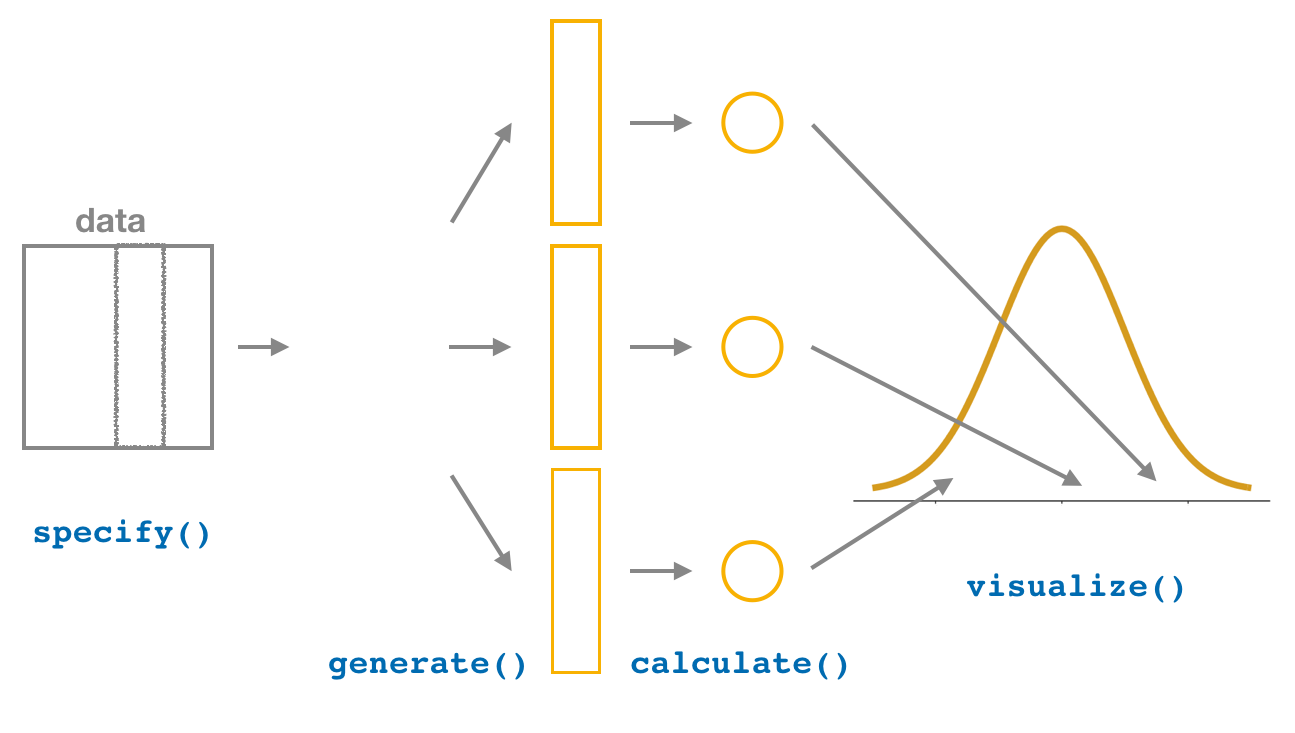FIGURE 9.8: Confidence intervals with the infer package.

In this section, we’ll now show you how to seamlessly modify the previously seen infer code for constructing confidence intervals to conduct hypothesis tests. You’ll notice that the basic outline of the workflow is almost identical, except for an additional hypothesize() step between the specify() and generate() steps, as can be seen in Figure 9.9.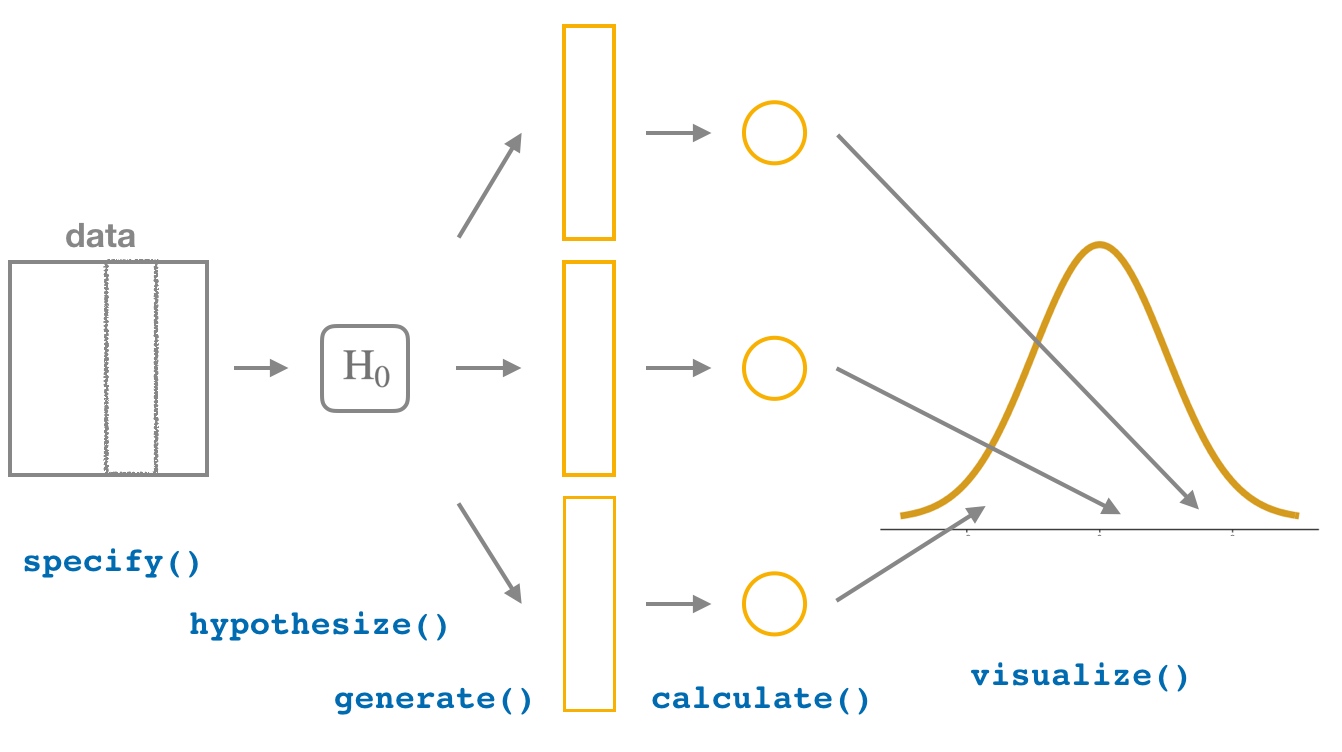FIGURE 9.9: Hypothesis testing with the infer package.

Furthermore, we’ll use a pre-specified significance level $$\alpha$$ = 0.05 for this hypothesis test. Let’s leave discussion on the choice of this $$\alpha$$ value until later on in Section 9.4.

### 9.3.1infer package workflow

#### 1. specify variables

Recall that we use the specify() verb to specify the response variable and, if needed, any explanatory variables for our study. In this case, since we are interested in any potential effects of gender on promotion decisions, we set decision as the response variable and gender as the explanatory variable. We do so using formula = response ~ explanatory where response is the name of the response variable in the data frame and explanatory is the name of the explanatory variable. So in our case it is decision ~ gender.

Furthermore, since we are interested in the proportion of résumés "promoted", and not the proportion of résumés not promoted, we set the argument success to "promoted".

promotions %>%
specify(formula = decision ~ gender, success = "promoted") 
Response: decision (factor)
Explanatory: gender (factor)
# A tibble: 48 x 2
decision gender
<fct>    <fct>
1 promoted male
2 promoted male
3 promoted male
4 promoted male
5 promoted male
6 promoted male
7 promoted male
8 promoted male
9 promoted male
10 promoted male
# … with 38 more rows

Again, notice how the promotions data itself doesn’t change, but the Response: decision (factor) and Explanatory: gender (factor) meta-data do. This is similar to how the group_by() verb from dplyr doesn’t change the data, but only adds “grouping” meta-data, as we saw in Section 3.4.

#### 2. hypothesize the null

In order to conduct hypothesis tests using the infer workflow, we need a new step not present for confidence intervals: hypothesize(). Recall from Section 9.2 that our hypothesis test was

\begin{aligned} H_0 &: p_{m} - p_{f} = 0\\ \text{vs. } H_A&: p_{m} - p_{f} > 0 \end{aligned}

In other words, the null hypothesis $$H_0$$ corresponding to our “hypothesized universe” stated that there was no difference in gender-based discrimination rates. We set this null hypothesis $$H_0$$ in our infer workflow using the null argument of the hypothesize() function to either:

• "point" for hypotheses involving a single sample or
• "independence" for hypotheses involving two samples.

In our case, since we have two samples (the résumés with “male” and “female” names), we set null = "independence".

promotions %>%
specify(formula = decision ~ gender, success = "promoted") %>%
hypothesize(null = "independence")
Response: decision (factor)
Explanatory: gender (factor)
Null Hypothesis: independence
# A tibble: 48 x 2
decision gender
<fct>    <fct>
1 promoted male
2 promoted male
3 promoted male
4 promoted male
5 promoted male
6 promoted male
7 promoted male
8 promoted male
9 promoted male
10 promoted male
# … with 38 more rows

Again, the data has not changed yet. This will occur at the upcoming generate() step; we’re merely setting meta-data for now.

Where do the terms "point" and "independence" come from? These are two technical statistical terms. The term “point” relates from the fact that for a single group of observations, you will test the value of a single point. Going back to the pennies example from Chapter 8, say we wanted to test if the mean year of all US pennies was equal to 1993 or not. We would be testing the value of a “point” $$\mu$$, the mean year of all US pennies, as follows

\begin{aligned} H_0 &: \mu = 1993\\ \text{vs } H_A&: \mu \neq 1993 \end{aligned}

The term “independence” relates to the fact that for two groups of observations, you are testing whether or not the response variable is independent of the explanatory variable that assigns the groups. In our case, we are testing whether the decision response variable is “independent” of the explanatory variable gender that assigns each résumé to either of the two groups.

#### 3. generate replicates

After we hypothesize() the null hypothesis, we generate() replicates of “shuffled” datasets assuming the null hypothesis is true. We do this by repeating the shuffling exercise you performed in Section 9.1 several times. Instead of merely doing it 16 times as our groups of friends did, let’s use the computer to repeat this 1000 times by setting reps = 1000 in the generate() function. However, unlike for confidence intervals where we generated replicates using type = "bootstrap" resampling with replacement, we’ll now perform shuffles/permutations by setting type = "permute". Recall that shuffles/permutations are a kind of resampling, but unlike the bootstrap method, they involve resampling without replacement.

promotions_generate <- promotions %>%
specify(formula = decision ~ gender, success = "promoted") %>%
hypothesize(null = "independence") %>%
generate(reps = 1000, type = "permute")
nrow(promotions_generate)
 48000

Observe that the resulting data frame has 48,000 rows. This is because we performed shuffles/permutations for each of the 48 rows 1000 times and $$48,000 = 1000 \cdot 48$$. If you explore the promotions_generate data frame with View(), you’ll notice that the variable replicate indicates which resample each row belongs to. So it has the value 1 48 times, the value 2 48 times, all the way through to the value 1000 48 times.

#### 4. calculate summary statistics

Now that we have generated 1000 replicates of “shuffles” assuming the null hypothesis is true, let’s calculate() the appropriate summary statistic for each of our 1000 shuffles. From Section 9.2, point estimates related to hypothesis testing have a specific name: test statistics. Since the unknown population parameter of interest is the difference in population proportions $$p_{m} - p_{f}$$, the test statistic here is the difference in sample proportions $$\widehat{p}_{m} - \widehat{p}_{f}$$.

For each of our 1000 shuffles, we can calculate this test statistic by setting stat = "diff in props". Furthermore, since we are interested in $$\widehat{p}_{m} - \widehat{p}_{f}$$ we set order = c("male", "female"). As we stated earlier, the order of the subtraction does not matter, so long as you stay consistent throughout your analysis and tailor your interpretations accordingly.

Let’s save the result in a data frame called null_distribution:

null_distribution <- promotions %>%
specify(formula = decision ~ gender, success = "promoted") %>%
hypothesize(null = "independence") %>%
generate(reps = 1000, type = "permute") %>%
calculate(stat = "diff in props", order = c("male", "female"))
null_distribution
# A tibble: 1,000 x 2
replicate       stat
<int>      <dbl>
1         1 -0.0416667
2         2 -0.125
3         3 -0.125
4         4 -0.0416667
5         5 -0.0416667
6         6 -0.125
7         7 -0.125
8         8 -0.125
9         9 -0.0416667
10        10 -0.0416667
# … with 990 more rows

Observe that we have 1000 values of stat, each representing one instance of $$\widehat{p}_{m} - \widehat{p}_{f}$$ in a hypothesized world of no gender discrimination. Observe as well that we chose the name of this data frame carefully: null_distribution. Recall once again from Section 9.2 that sampling distributions when the null hypothesis $$H_0$$ is assumed to be true have a special name: the null distribution.

What was the observed difference in promotion rates? In other words, what was the observed test statistic $$\widehat{p}_{m} - \widehat{p}_{f}$$? Recall from Section 9.1 that we computed this observed difference by hand to be 0.875 - 0.583 = 0.292 = 29.2%. We can also compute this value using the previous infer code but with the hypothesize() and generate() steps removed. Let’s save this in obs_diff_prop:

obs_diff_prop <- promotions %>%
specify(decision ~ gender, success = "promoted") %>%
calculate(stat = "diff in props", order = c("male", "female"))
obs_diff_prop
# A tibble: 1 x 1
stat
<dbl>
1 0.291667

#### 5. visualize the p-value

The final step is to measure how surprised we are by a promotion difference of 29.2% in a hypothesized universe of no gender discrimination. If the observed difference of 0.292 is highly unlikely, then we would be inclined to reject the validity of our hypothesized universe.

We start by visualizing the null distribution of our 1000 values of $$\widehat{p}_{m} - \widehat{p}_{f}$$ using visualize() in Figure 9.10. Recall that these are values of the difference in promotion rates assuming $$H_0$$ is true. This corresponds to being in our hypothesized universe of no gender discrimination.

visualize(null_distribution, bins = 10)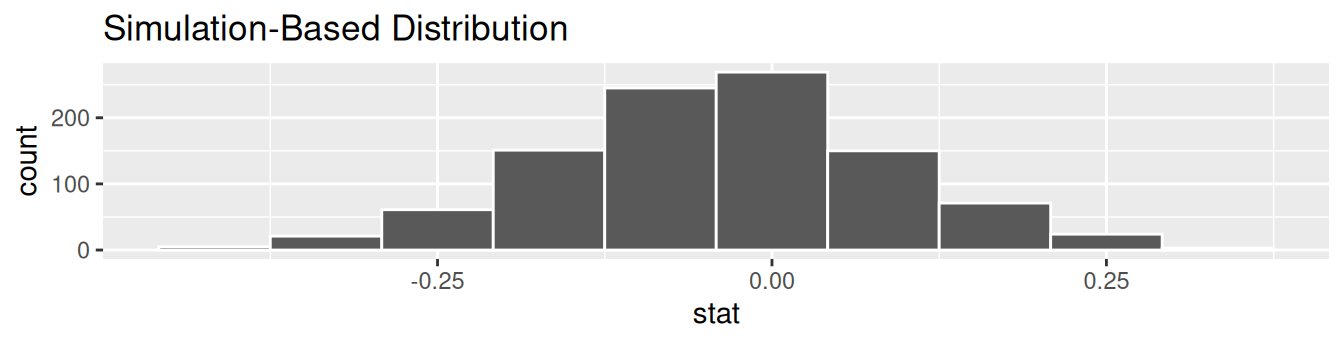FIGURE 9.10: Null distribution.

Let’s now add what happened in real life to Figure 9.10, the observed difference in promotion rates of 0.875 - 0.583 = 0.292 = 29.2%. However, instead of merely adding a vertical line using geom_vline(), let’s use the shade_p_value() function with obs_stat set to the observed test statistic value we saved in obs_diff_prop.

Furthermore, we’ll set the direction = "right" reflecting our alternative hypothesis $$H_A: p_{m} - p_{f} > 0$$. Recall our alternative hypothesis $$H_A$$ is that $$p_{m} - p_{f} > 0$$, stating that there is a difference in promotion rates in favor of résumés with male names. “More extreme” here corresponds to differences that are “bigger” or “more positive” or “more to the right.” Hence we set the direction argument of shade_p_value() to be "right".

On the other hand, had our alternative hypothesis $$H_A$$ been the other possible one-sided alternative $$p_{m} - p_{f} < 0$$, suggesting discrimination in favor of résumés with female names, we would’ve set direction = "left". Had our alternative hypothesis $$H_A$$ been two-sided $$p_{m} - p_{f} \neq 0$$, suggesting discrimination in either direction, we would’ve set direction = "both".

visualize(null_distribution, bins = 10) +
shade_p_value(obs_stat = obs_diff_prop, direction = "right")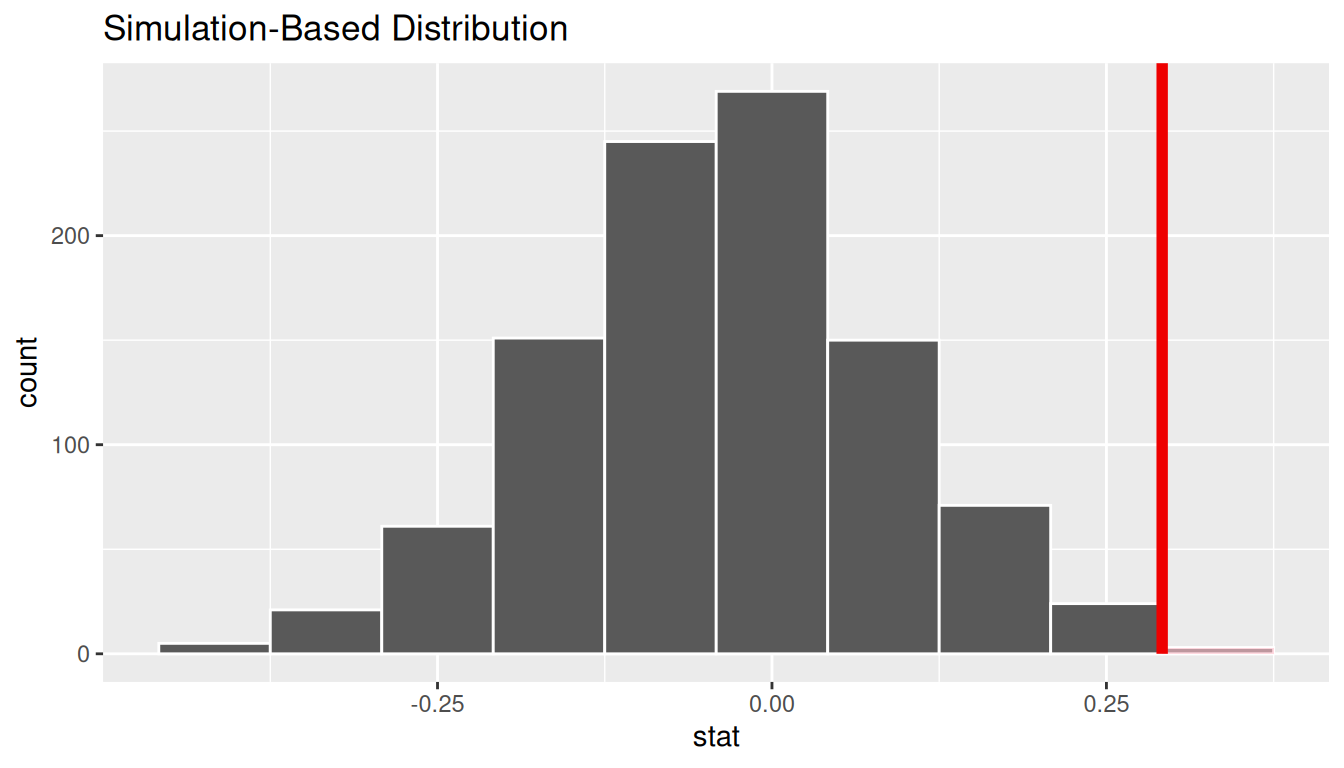FIGURE 9.11: Shaded histogram to show $$p$$-value.

In the resulting Figure 9.11, the solid dark line marks 0.292 = 29.2%. However, what does the shaded-region correspond to? This is the $$p$$-value. Recall the definition of the $$p$$-value from Section 9.2:

A $$p$$-value is the probability of obtaining a test statistic just as or more extreme than the observed test statistic assuming the null hypothesis $$H_0$$ is true.

So judging by the shaded region in Figure 9.11, it seems we would somewhat rarely observe differences in promotion rates of 0.292 = 29.2% or more in a hypothesized universe of no gender discrimination. In other words, the $$p$$-value is somewhat small. Hence, we would be inclined to reject this hypothesized universe, or using statistical language we would “reject $$H_0$$.”

What fraction of the null distribution is shaded? In other words, what is the exact value of the $$p$$-value? We can compute it using the get_p_value() function with the same arguments as the previous shade_p_value() code:

null_distribution %>%
get_p_value(obs_stat = obs_diff_prop, direction = "right")
# A tibble: 1 x 1
p_value
<dbl>
1   0.027

Keeping the definition of a $$p$$-value in mind, the probability of observing a difference in promotion rates as large as 0.292 = 29.2% due to sampling variation alone in the null distribution is 0.027 = 2.7%. Since this $$p$$-value is smaller than our pre-specified significance level $$\alpha$$ = 0.05, we reject the null hypothesis $$H_0: p_{m} - p_{f} = 0$$. In other words, this $$p$$-value is sufficiently small to reject our hypothesized universe of no gender discrimination. We instead have enough evidence to change our mind in favor of gender discrimination being a likely culprit here. Observe that whether we reject the null hypothesis $$H_0$$ or not depends in large part on our choice of significance level $$\alpha$$. We’ll discuss this more in Subsection 9.4.3.

### 9.3.2 Comparison with confidence intervals

One of the great things about the infer package is that we can jump seamlessly between conducting hypothesis tests and constructing confidence intervals with minimal changes! Recall the code from the previous section that creates the null distribution, which in turn is needed to compute the $$p$$-value:

null_distribution <- promotions %>%
specify(formula = decision ~ gender, success = "promoted") %>%
hypothesize(null = "independence") %>%
generate(reps = 1000, type = "permute") %>%
calculate(stat = "diff in props", order = c("male", "female"))

To create the corresponding bootstrap distribution needed to construct a 95% confidence interval for $$p_{m} - p_{f}$$, we only need to make two changes. First, we remove the hypothesize() step since we are no longer assuming a null hypothesis $$H_0$$ is true. We can do this by deleting or commenting out the hypothesize() line of code. Second, we switch the type of resampling in the generate() step to be "bootstrap" instead of "permute".

bootstrap_distribution <- promotions %>%
specify(formula = decision ~ gender, success = "promoted") %>%
# Change 1 - Remove hypothesize():
# hypothesize(null = "independence") %>%
# Change 2 - Switch type from "permute" to "bootstrap":
generate(reps = 1000, type = "bootstrap") %>%
calculate(stat = "diff in props", order = c("male", "female"))

Using this bootstrap_distribution, let’s first compute the percentile-based confidence intervals, as we did in Section 8.4:

percentile_ci <- bootstrap_distribution %>%
get_confidence_interval(level = 0.95, type = "percentile")
percentile_ci
# A tibble: 1 x 2
2.5%  97.5%
<dbl>    <dbl>
1 0.0444444 0.538542

Using our shorthand interpretation for 95% confidence intervals from Subsection 8.5.2, we are 95% “confident” that the true difference in population proportions $$p_{m} - p_{f}$$ is between (0.044, 0.539). Let’s visualize bootstrap_distribution and this percentile-based 95% confidence interval for $$p_{m} - p_{f}$$ in Figure 9.12.

visualize(bootstrap_distribution) +
shade_confidence_interval(endpoints = percentile_ci)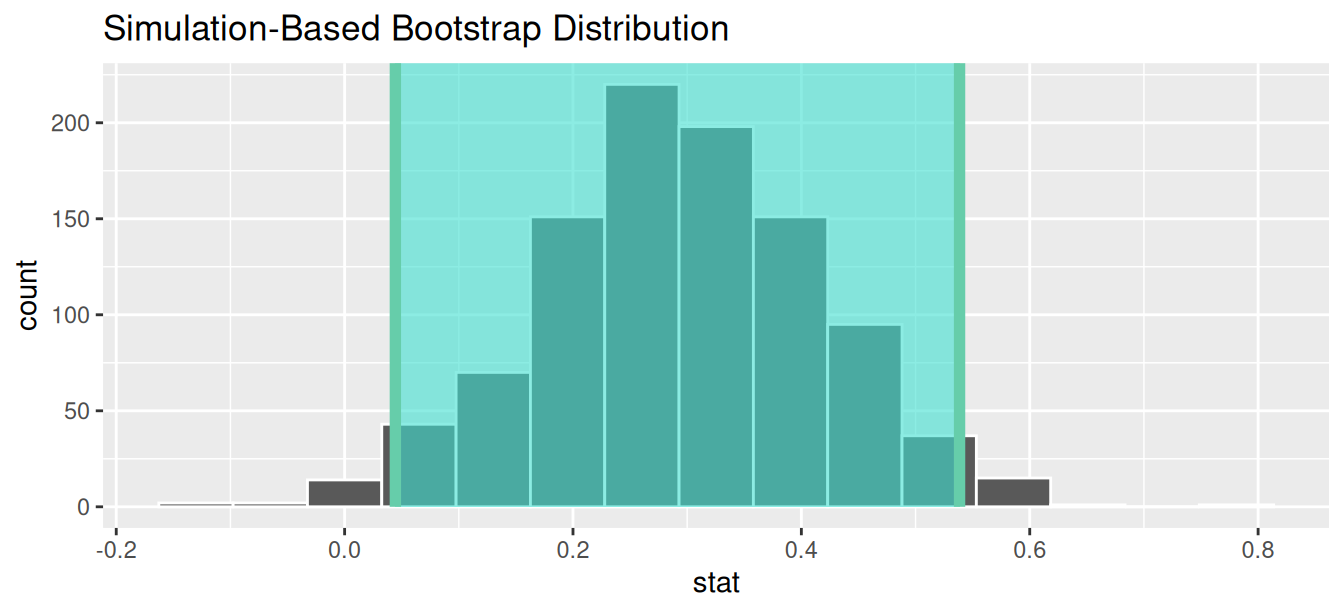FIGURE 9.12: Percentile-based 95% confidence interval.

Notice a key value that is not included in the 95% confidence interval for $$p_{m} - p_{f}$$: the value 0. In other words, a difference of 0 is not included in our net, suggesting that $$p_{m}$$ and $$p_{f}$$ are truly different! Furthermore, observe how the entirety of the 95% confidence interval for $$p_{m} - p_{f}$$ lies above 0, suggesting that this difference is in favor of men.

Since the bootstrap distribution appears to be roughly normally shaped, we can also use the standard error method as we did in Section 8.4. In this case, we must specify the point_estimate argument as the observed difference in promotion rates 0.292 = 29.2% saved in obs_diff_prop. This value acts as the center of the confidence interval.

se_ci <- bootstrap_distribution %>%
get_confidence_interval(level = 0.95, type = "se",
point_estimate = obs_diff_prop)
se_ci
# A tibble: 1 x 2
lower    upper
<dbl>    <dbl>
1 0.0514129 0.531920

Let’s visualize bootstrap_distribution again, but now the standard error based 95% confidence interval for $$p_{m} - p_{f}$$ in Figure 9.13. Again, notice how the value 0 is not included in our confidence interval, again suggesting that $$p_{m}$$ and $$p_{f}$$ are truly different!

visualize(bootstrap_distribution) +
shade_confidence_interval(endpoints = se_ci)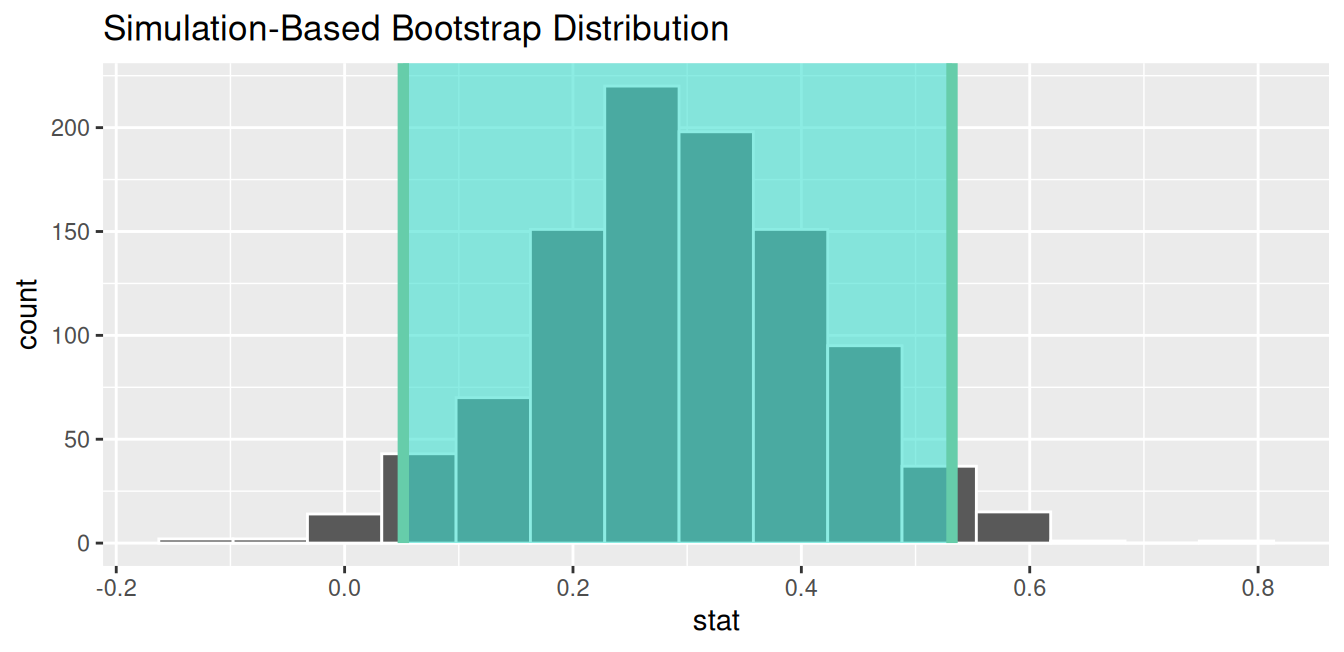FIGURE 9.13: Standard error-based 95% confidence interval.

Learning check

(LC9.1) Conduct the same hypothesis test and confidence interval analysis comparing male and female promotion rates using the median rating instead of the mean rating. What was different and what was the same?

(LC9.2) Why are we relatively confident that the distributions of the sample proportions will be good approximations of the population distributions of promotion proportions for the two genders?

(LC9.3) Using the definition of p-value, write in words what the $$p$$-value represents for the hypothesis test comparing the promotion rates for males and females.

### 9.3.3 “There is only one test”

Let’s recap the steps necessary to conduct a hypothesis test using the terminology, notation, and definitions related to sampling you saw in Section 9.2 and the infer workflow from Subsection 9.3.1:

1. specify() the variables of interest in your data frame.
2. hypothesize() the null hypothesis $$H_0$$. In other words, set a “model for the universe” assuming $$H_0$$ is true.
3. generate() shuffles assuming $$H_0$$ is true. In other words, simulate data assuming $$H_0$$ is true.
4. calculate() the test statistic of interest, both for the observed data and your simulated data.
5. visualize() the resulting null distribution and compute the $$p$$-value by comparing the null distribution to the observed test statistic.

While this is a lot to digest, especially the first time you encounter hypothesis testing, the nice thing is that once you understand this general framework, then you can understand any hypothesis test. In a famous blog post, computer scientist Allen Downey called this the “There is only one test” framework, for which he created the flowchart displayed in Figure 9.14.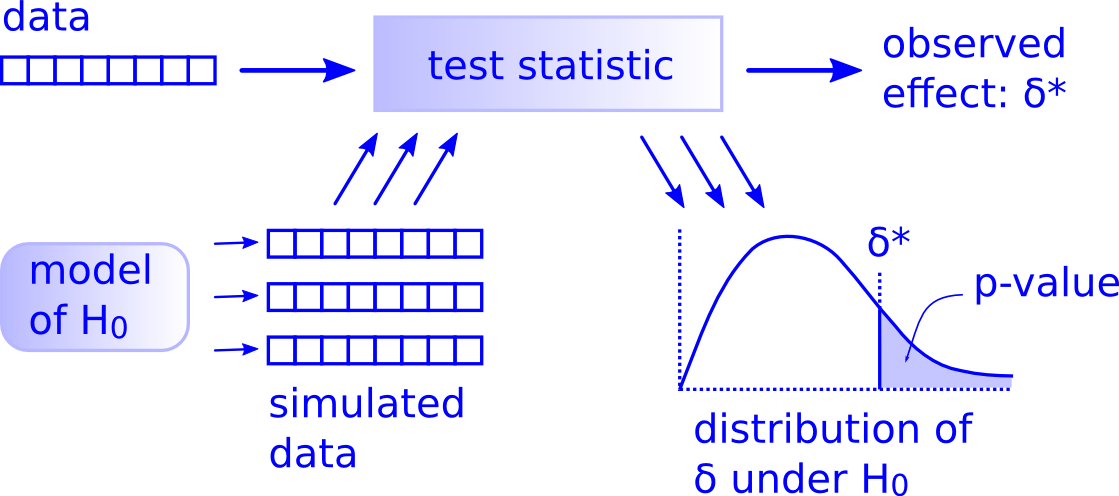FIGURE 9.14: Allen Downey’s hypothesis testing framework.

Notice its similarity with the “hypothesis testing with infer” diagram you saw in Figure 9.9. That’s because the infer package was explicitly designed to match the “There is only one test” framework. So if you can understand the framework, you can easily generalize these ideas for all hypothesis testing scenarios. Whether for population proportions $$p$$, population means $$\mu$$, differences in population proportions $$p_1 - p_2$$, differences in population means $$\mu_1 - \mu_2$$, and as you’ll see in Chapter 10 on inference for regression, population regression slopes $$\beta_1$$ as well. In fact, it applies more generally even than just these examples to more complicated hypothesis tests and test statistics as well.

Learning check

(LC9.4) Describe in a paragraph how we used Allen Downey’s diagram to conclude if a statistical difference existed between the promotion rate of males and females using this study.